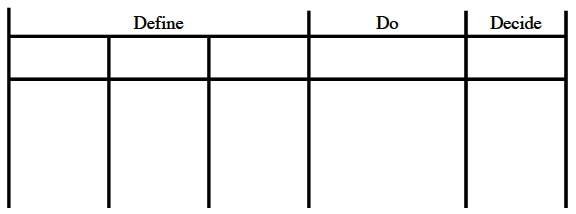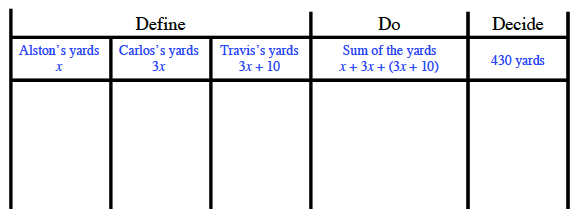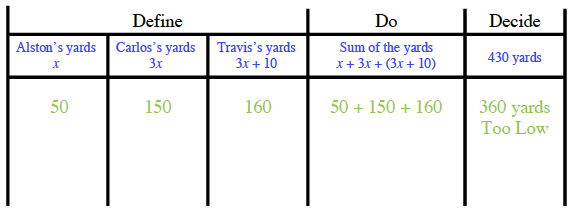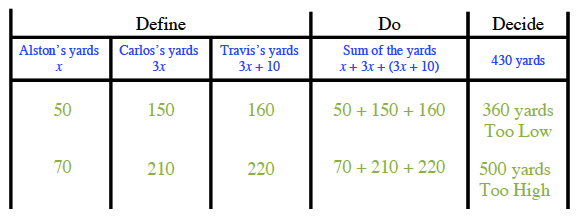### Home > CC2 > Chapter Ch6 > Lesson 6.2.2 > Problem6-70

6-70.

To solve the following problem, use the 5-D Process. Define a variable and write an expression for each column of your table.

In the first three football games of the season, Carlos gained three times as many yards as Alston. Travis gained ten yards more than Carlos. Altogether, the three players gained a total of $430$ yards. How many yards did Carlos gain?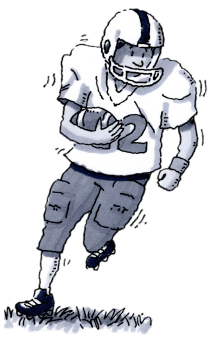Based on the information from the problem, two of the players, Carlos and Travis, can be directly related to Alston in yardage. If Alston's yards are represented by the variable, $x$, how can Carlos and Travis' yards be represented? A 5 column table, first row labeled as follows: First 3 columns, define, fourth, do, fifth, decide.

Row 2 labels added as follows: Define 1: Alston's yards, x. Define 2: Carlo's yards, 3 x. Define 3: Carlos's yards, 3 x + 10. Do: Sum of the yards, x + 3, x + 3, x + 10. Decide: 430 yards.

Row 3 labels, left to right: 50, 150, 160, 50 + 150 + 160, 360 yards too low.

Now run some trials of your own to determine how many yards Carlos gained. Row 4 labels, left to right: 70, 210, 220, 70 + 210 + 220, 500 yards too high.